# PSEB 7th Class Maths Solutions Chapter 1 Integers Ex 1.4

Punjab State Board PSEB 7th Class Maths Book Solutions Chapter 1 Integers Ex 1.4 Textbook Exercise Questions and Answers.

## PSEB Solutions for Class 7 Maths Chapter 1 Integers Ex 1.4

1. Evaluate each of the following :
(i) 76 ÷ 19
(ii) (-156) ÷ (-12)
(iii) (-125) ÷ (-1)
(iv) (125) ÷ (-25)
(v) 0 ÷ (-5)
(vi) (-15) ÷ (15)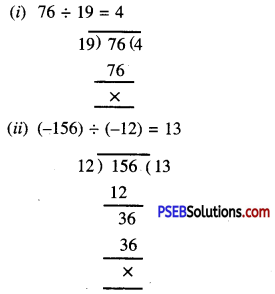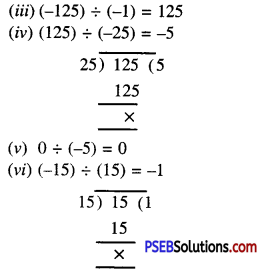2. Write all even integers between -18 and 0.
All even integers between – 18 and 0 are :
-16, -14, -12, -10, -8, -6, -4, -2.

3. Write all odd integers between -9 and 9.
All odd integers between -9 and 9 are :
-7, -5, -3, -1, 1, 3, 5, 7.4. By what number should (-240) be divided to obtain 16.
Let the required number be x
∴ -240 ÷ x = 16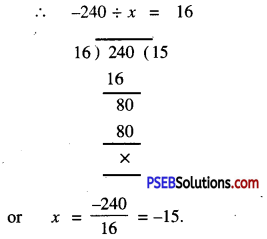Hence, the required number is -15

5. Find the value of :

Question (i).
125 ÷ [5 ÷ (-1)]
125 ÷ [5 ÷ (-1)] = 125 ÷ (-5)
= -25 Ans.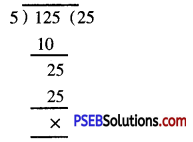Question (ii).
[169 ÷ 13] ÷ [26 ÷ 2]
[169 ÷ 13] ÷ [26 ÷ 2]
=  ÷  = 1 Ans.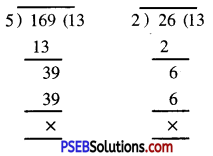Question (iii).
[(-105) ÷ 3] ÷ 7
[(-105) ÷ 3] ÷ 7
= [-35] ÷ 7
= -5.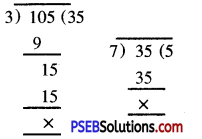6. Simplify : 12 – [8 + 27 ÷ (2 × 8 – 7)]
12 – [8 + 27 + (2 × 8 – 7)]
= 12 – [8 + 27 ÷ (16 – 7)]
= 12 – [8 + 27 ÷ (9)]
= 12 – [8 + 3] = 12- 11
= 17. Simplify : 10 – [8 – {11 + 30 ÷ (4 + 2)}]
10 – [8 – {11 + 30 (4 + 2)}]
= 10 – [8 – {11 + 30 ÷ 6}]
= 10 – [8 – (11 + 5)]
= 10 – [8 – 16]
= 10 – [-8]
= 10 + 8 = 18

8. Multiple Choice Questions :

Question (i).
(-8) ÷ 2 =
(a) -16
(b) -4
(c) 4
(d) -8.
(b) -4

Question (ii).
(-7) ÷ (-7) =
(a) -1
(b) 49
(c) -49
(d) None of these.
(d) None of these.

Question (iii).
0 ÷ 2 =
(a) 1
(b) 2
(c) -2
(d) 0.